﻿ 先进战斗机强度设计技术发展与实践Download PDF文章快速检索 高级检索

Development and application of strength design technology of high performance fighter
ZHANG Lixin, ZHONG Shunlu, LIU Xiaodong, FU Huanbing, DUI Hongna, LIU Dongliang, JING Luyun, MOU Binjie, SHI Shanglu
Department of Strength, AVIC Chengdu Aircraft Design and Research Institute, Chengdu 610091, China
Abstract: The airframe of high performance fighter requires light weight, long life, multi-function, and high g capacity. To achieve this goal, in addition to the contribution of materials and manufacturing technology (new materials, new processes, and new structures), structural strength design, analysis, and verification technology of design institute must also be improved to meet the requirements. Focusing on the requirements of structural integrity, this paper describes the research results, technical development, and design practice of the strength team in recent years. It mainly includes the specification architecture for strength design and verification of high performance fighter, structural load screening technology based on multi-dimensional envelope, internal loads analysis technology based on unified FEM model, composite integrated structure analysis technology, detail stress analysis technology with high precision and efficiency, pre-tightening principle and strength design of embedded weapon bay door, rapid modeling, and acoustic fatigue analysis method of curved stiffened panel, prognostic and health management system design. The above research results have been successfully applied to the development of a new generation of high performance fighter airframe.
Keywords: strength specification architecture    load screening    internal loads analysis    composite integrated structure analysis    detailed stress analysis    embedded weapon bay pre-tightening door    acoustic fatigue    prognostic and health management

F-16飞机在寿命期内贯彻结构完整性大纲堪称典范，在项目执行和技术方面的杰出成就主要来自于高效的协同、稳健的技术规范架构/体系，共享的结构设计/分析/维护数据库、高效的数据处理等。高保真的结构有限元模型与强度分析/验证技术是贯彻执行结构完整性大纲的基础，F-35飞机在不同阶段构建了不同成熟度的有限元模型，最终构建经地面试验和飞行试验确认/验证的能高度代表机体的模型，用于结构完整性大纲规定的所有结构强度分析与验证[5-6]

1 面向新一代先进战斗机强度设计与验证的规范架构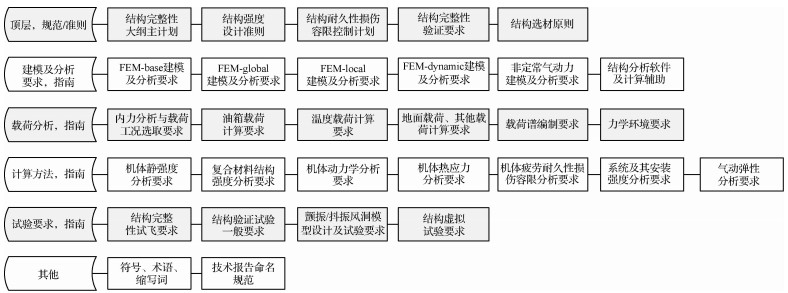图 1 面向新一代先进战斗机强度设计与验证的规范架构 Fig. 1 Specification architecture for strength design and verification of high performance fighter
2 基于多维包线的结构载荷筛选技术

1) 对组合包线应用于飞机强度校核各工程量的合理性进行了论证。

2) 将二内力组合包线的表达式推广到多内力组合，并结合机体结构特点对表达式进行了工程简化。

3) 结合板壳理论将传统基于一维梁的内力扩展到基于二维板壳的内力张量。

4) 在理论推导的基础上完成了相关程序的编写并在新型号飞机强度设计上完成了应用，抓住了常规载荷筛选方法容易遗漏的工况，同时减少载荷设计工况90%以上，极大促进了强度设计工作的高效准确完成。

2.1 包线原理对强度工程量的适用性

 $\left\{ {\begin{array}{*{20}{l}} {\mathit{\boldsymbol{Y}}(k\mathit{\boldsymbol{F}}) = k\mathit{\boldsymbol{Y}}(\mathit{\boldsymbol{F}})}\\ {\mathit{\boldsymbol{Y}}(a{\mathit{\boldsymbol{F}}_{\rm{A}}} + b{\mathit{\boldsymbol{F}}_{\rm{B}}}) = a\mathit{\boldsymbol{Y}}({\mathit{\boldsymbol{F}}_{\rm{A}}}) + b\mathit{\boldsymbol{Y}}({\mathit{\boldsymbol{F}}_{\rm{B}}})} \end{array}} \right.$ （1）

 $\left\{ {\begin{array}{*{20}{l}} {{\rm{min}}({Y^\prime }({\mathit{\boldsymbol{F}}_{\rm{A}}}),{Y^\prime }({\mathit{\boldsymbol{F}}_{\rm{B}}})) \le {Y^\prime }(a{\mathit{\boldsymbol{F}}_{\rm{A}}} + (1 - a){\mathit{\boldsymbol{F}}_{\rm{B}}})}\\ {{Y^\prime }(a{\mathit{\boldsymbol{F}}_{\rm{A}}} + (1 - a){\mathit{\boldsymbol{F}}_{\rm{B}}}) \le {\rm{max}}({Y^\prime }({\mathit{\boldsymbol{F}}_{\rm{A}}}),{Y^\prime }({\mathit{\boldsymbol{F}}_{\rm{B}}}))} \end{array}} \right.$ （2）

 $\left\{ {\begin{array}{*{20}{l}} {{\rm{min}}({Y^\prime }({\mathit{\boldsymbol{F}}_{\rm{A}}}),Y({\mathit{\boldsymbol{F}}_{\rm{B}}})) \le {Y^\prime }(a{\mathit{\boldsymbol{F}}_{\rm{A}}} + b{\mathit{\boldsymbol{F}}_{\rm{B}}})}\\ {{Y^\prime }(a{\mathit{\boldsymbol{F}}_{\rm{A}}} + b{\mathit{\boldsymbol{F}}_{\rm{B}}}) \le {\rm{max}}({Y^\prime }({\mathit{\boldsymbol{F}}_{\rm{A}}}),{Y^\prime }({\mathit{\boldsymbol{F}}_{\rm{B}}}))} \end{array}} \right.$ （3）

2.2 基于一维梁的多维包线及工程化处理

 $\mathit{\boldsymbol{F}} = \sum\limits_{i = 1}^n {{a_i}} {\mathit{\boldsymbol{F}}_i}{\kern 1pt} {\kern 1pt} {\kern 1pt} {\kern 1pt} {\kern 1pt} {\kern 1pt} {\kern 1pt} {\kern 1pt} {\kern 1pt} {\kern 1pt} {\kern 1pt} {\kern 1pt} {\kern 1pt} {\kern 1pt} 0 \le {a_i},\sum\limits_{i = 1}^n {{a_i}} \le 1$ （4）

F对结构不构成新的严重工况。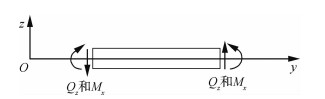图 2 纵向内力正方向定义 Fig. 2 Definition of positive direction of longitudinal internal force

 $\left\{ \begin{array}{l} {Q_{z{\rm{A}}}} < {Q_{z{\rm{B}}}},{Q_{x{\rm{A}}}} < {Q_{x{\rm{B}}}},{M_{y{\rm{A}}}} < {M_{y{\rm{B}}}}\\ \left| {{M_{x{\rm{A}}}}} \right| < \left| {{M_{x{\rm{B}}}}} \right|{\kern 1pt} {\kern 1pt} {\kern 1pt} {\rm{且同号}}{\kern 1pt} {\kern 1pt} {\rm{,}}{M_{z{\rm{A}}}} < {M_{z{\rm{B}}}} \end{array} \right.$ （5）

2.3 基于板壳理论的内力张量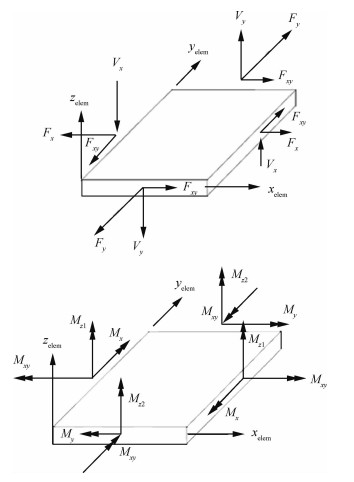图 3 二维单元内力图 Fig. 3 Internal force for two dimensional element

3 基于统一模型的全机内力分析技术

3.1 全机统一建模

 载荷类型 ROD BAR BEAM MEMBR BENDING SHEAR SHELL 拉 √ √ 〇 √ × 〇 √ 压 √ √ 〇 〇 × × √ 弯 × √ √ × √ × √ 剪 × √ √ × 〇 √ √ 扭 〇 √ √ × 〇 × √ 面外压 × × × 〇 〇 × √ 注：√表示完全适用，〇表示部分适用或有一定功能限制，×表示不适用。对于机身或长直翼类结构的截面刚度、截面内力分析，使用一种专用的组合结构工程梁截面模型，典型模型见图 4。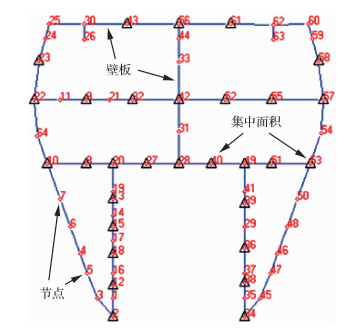图 4 典型工程梁截面模型 Fig. 4 Typical section model of engineering beam

 单元 属性参数 工程梁模型 统一有限元模型 点单元 类型 POINT ROD/BAR/BEAM 弹性模量(系数) Ei/E0 E=Ei 集中面积 Area Area=Ai 壁单元 类型 PANEL MEMBR/BENDING/SHEAR/SHELL 弹性模量(系数) Ei/E0 E=Ei 厚度 Ti T=Ti

3.2 内力分析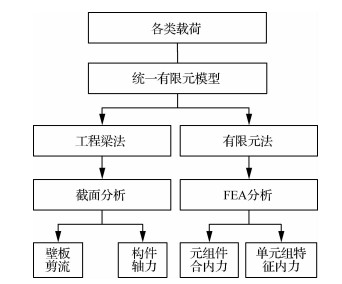图 5 结构内力分析方法和流程简图 Fig. 5 Structural internal force analysis method and flow diagram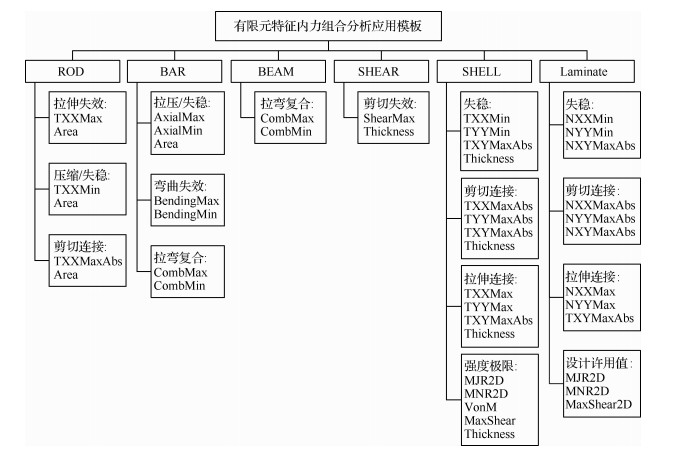图 6 典型结构有限元特征内力组合分析 Fig. 6 Internal force analysis of finite element characteristic for typical structures

 ${\rm{MS}} = \frac{{[P]}}{P} - 1 > 0$ （6）

 ${\rm{MS}} = \frac{{[P_i^ * ]}}{{{P_i}}} - 1$ （7）

 $f\left( {\frac{{{P_1}}}{{[{P_1}]}},\frac{{{P_2}}}{{[{P_2}]}}, \cdots } \right) = 1$ （8）

 ${\rm{min}}\left( {\frac{{[P_i^ * ]}}{{{P_i}}}} \right) \ge 1$ （9）

3.3 结构分析流程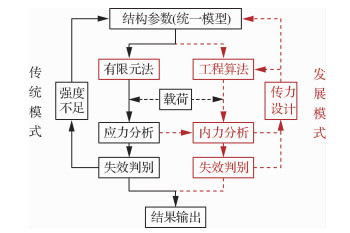图 7 结构强度设计分析流程 Fig. 7 Structural strength design and analysis process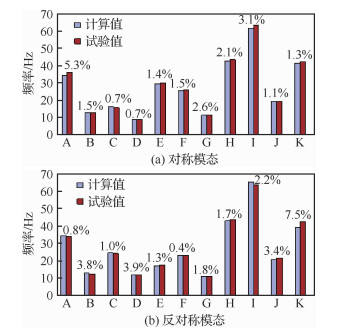图 8 GVT试验与仿真分析结果对比 Fig. 8 Comparison of GVT test and simulation analysis results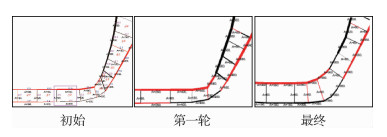图 9 主承力框加强筋参数迭代过程 Fig. 9 Parameter iterations process of main frame stiffeners

4 复合材料整体化结构分析技术

4.1 结构单元界面分析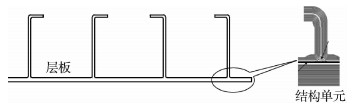图 10 整体化结构单元 Fig. 10 Integrated structure unit

 ${\left( {\frac{{{t_{\rm{n}}}}}{{t_{\rm{n}}^0}}} \right)^2} + {\left( {\frac{{{t_{\rm{s}}}}}{{t_{\rm{s}}^0}}} \right)^2} + {\left( {\frac{{{t_{\rm{t}}}}}{{t_{\rm{t}}^0}}} \right)^2} = 1$ （10）

 ${G_{\rm{c}}} = {G_{{\rm{Ic}}}} + ({G_{{\rm{IIc}}}} - {G_{{\rm{Ic}}}}) \cdot {\left( {\frac{{{G_{\rm{S}}}}}{{{G_{\rm{T}}}}}} \right)^\eta }$ （11）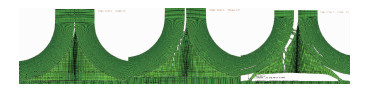图 11 U型结构单元界面失效过程仿真 Fig. 11 Simulation of interface failure process of structure unit of U shape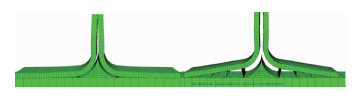图 12 缝纫增强结构单元界面失效过程仿真 Fig. 12 Simulation of interface failure process of sewing reinforced structure unit

4.2 结构单元特征参数表征及影响分析

4.2.1 界面材料(胶粘剂)强度对极限载荷的影响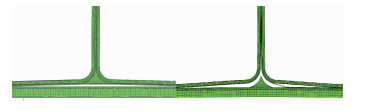图 13 不同界面材料失效模式 Fig. 13 Failure modes of materials on different interfaces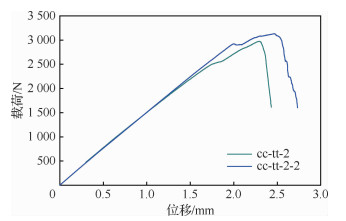图 14 界面材料对拉伸载荷-位移曲线的影响 Fig. 14 Effect of interface materials on tensile load-displacement curve
4.2.2 三角区填料刚度对强度的影响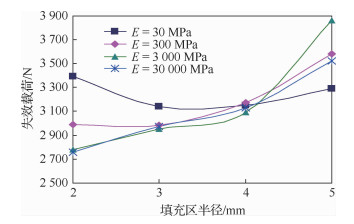图 15 拉伸失效载荷与填料弹性模量的关系 Fig. 15 Tensile failure load and elastic modulus variation curves of packing in filling zone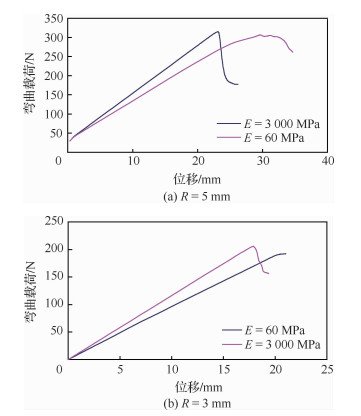图 16 填充区不同填料弹性模量的弯曲载荷-位移曲线 Fig. 16 Bending load-displacement curves with different elastic modulus in filling zone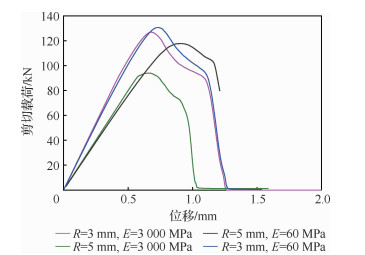图 17 填充区不同填料弹性模量的剪切载荷-位移曲线 Fig. 17 Shearing load-displacement curves with different elastic modulus in filling zone
4.2.3 界面填充区半径对承载能力的影响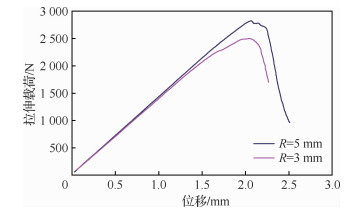图 18 不同填充区半径的拉伸载荷-位移曲线 Fig. 18 Tensile load-displacement curves with different filling zone radius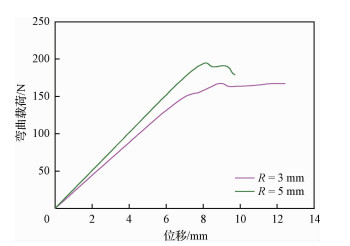图 19 不同填充区半径的弯曲载荷-位移曲线 Fig. 19 Bending load-displacement curves of different filling zone radius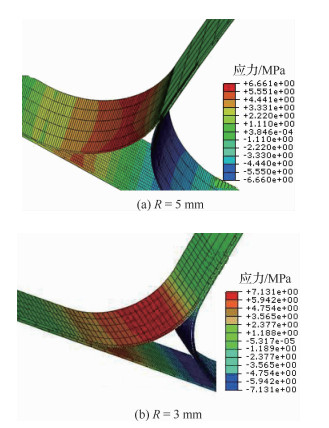图 20 R区层间应力 Fig. 20 Interlaminar stress of section R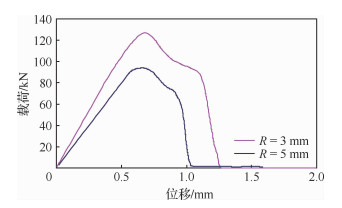图 21 不同填充区半径的剪切载荷-位移曲线 Fig. 21 Shear-displacement curves of different filling zone radius
4.2.4 缝纫参数对缝合整体壁板的影响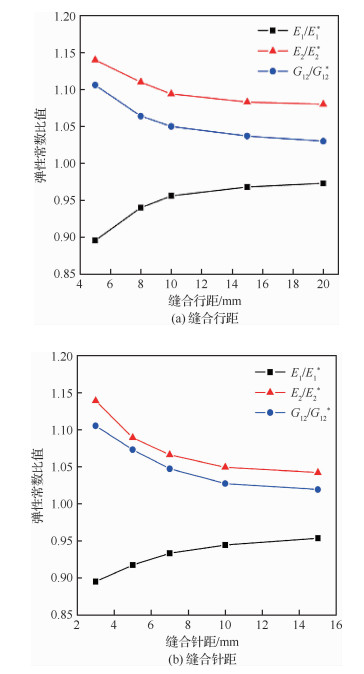图 22 缝合参数对层板面内模量的影响 Fig. 22 Effect of stitching parameters on in-plane modulus of laminate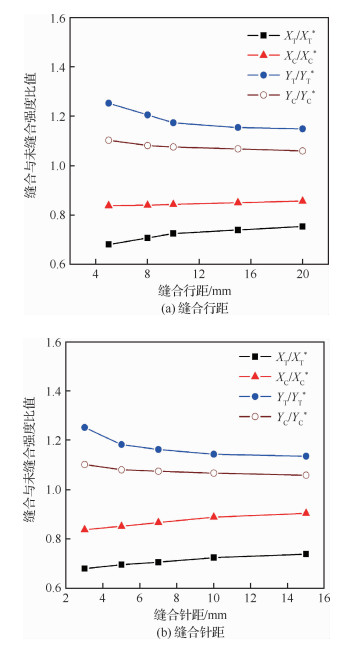图 23 缝合参数对层板强度的影响 Fig. 23 Effect of stitching parameters on strength of laminate

5 高精度快速细节应力分析技术 5.1 细节应力分析

1) 直接细化法，即选取适当边界，直接在总体模型中建立局部细节细化模型，并采用适当方式处理细化区域与周边的连接，以实现载荷与位移协调。

2) 刚度缩聚法，包括子结构法和直接矩阵输入技术(Direct Input Matrix at Grid points，DIMG)。

3) Global-Local法，在总体分析模型中选取适当边界，将局部细节从总体模型中取出形成自由体(Free Body)，施加总体模型中局部细节与总体模型交界边界条件(位移或力)，重新求解。

5.2 基于力边界的Global-Local方法

Global-Local分析方法有力边界或位移边界2种方式，本文主要介绍主要基于力边界的Global-Local方法。

5.2.1 基本原理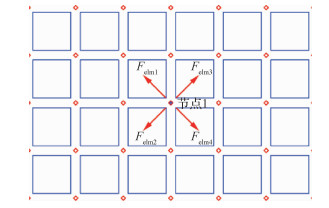图 24 节点平衡力概念 Fig. 24 Concept of node balance force

5.2.2 基本流程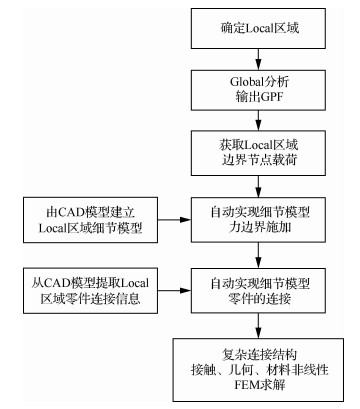图 25 基于力法的Global-Local细节应力分析流程 Fig. 25 Flow chart of Global-Local detail stress analysis based on force method
5.3 刚度差异影响及处理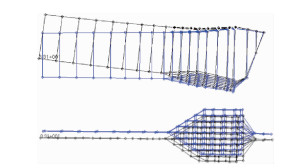图 26 总体分析中梁的变形 Fig. 26 Deformation of beam in global analysis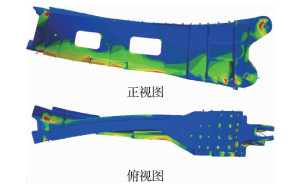图 27 梁细节应力最大主应力云图(含变形) Fig. 27 The max principal stress contour of a beam in detail analysis (with deformation)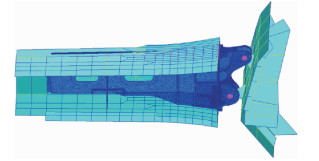图 28 实体模型替代板杆元模型 Fig. 28 Replacing shell-rod elements model with solid elements model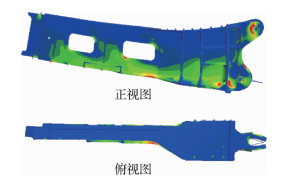图 29 改进后梁细节应力分析最大主应力云图(含变形) Fig. 29 The max principal stress contour of a beam in modified detail analysis (with deformation)

 框 交点 偏差/% 原状态 状态1 状态2 状态3 状态4 状态5 框1 左上 0 3.422 1.325 1.419 0.970 0.970 左下 0 3.761 1.728 1.264 0.853 0.853 右上 0 4.753 1.523 1.479 0.948 0.948 右下 0 3.725 1.869 1.300 0.826 0.826 框2 左上 0 0.767 1.652 1.380 0.940 0.940 左下 0 0.838 1.783 2.218 1.438 1.438 右上 0 0.097 1.442 1.392 0.884 0.884 右下 0 0.806 1.684 2.298 1.416 1.416 框3 左上 0 -0.887 -0.245 -0.052 1.199 1.199 左下 0 -0.759 -0.156 -0.421 2.028 2.028 右上 0 -1.480 -0.733 -0.608 1.060 1.060 右下 0 -1.095 -0.469 -0.818 2.019 2.019 框4 左上 0 -3.968 -3.535 -3.466 -3.602 -3.602 左下 0 -5.490 -5.069 -5.093 -5.673 -5.673 右上 0 -3.445 -3.006 -3.000 -3.305 -3.305 右下 0 -5.273 -4.809 -4.894 -5.604 -5.604 注：①原状态：4个框为板杆单元；②状态1~状态4中实体单元为粗网格，一阶单元(平均尺寸10 mm)；③状态1：4个框均为实体单元；④状态2：框2~框4为实体单元；⑤状态3：框3、框4为实体单元；⑥状态4：框4为实体单元；⑦状态5：框4为实体单元(细网格，平均尺寸5 mm，一阶单元)。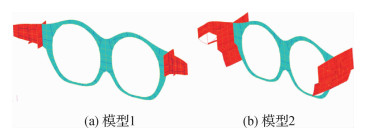图 30 分析模型的不同边界条件选取 Fig. 30 Different boundary conditions selected for analysis model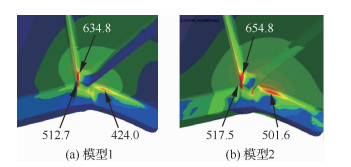图 31 不同边界条件最大正应力结果对比 Fig. 31 Comparison of max principal stress results with different boundary condition
5.4 自动分层连接技术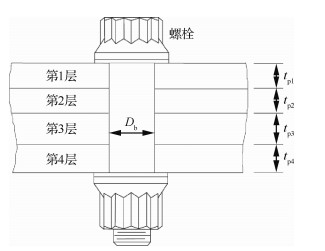图 32 典型螺栓连接 Fig. 32 Classic bolt connecter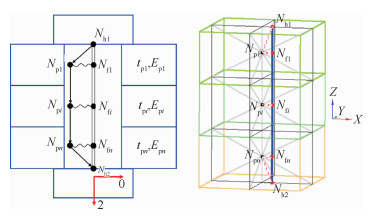图 33 模拟连接 Fig. 33 Simulant connector

5.5 边界外载与边界MPC处理技术

5.5.1 边界上有集中载荷

5.5.2 有跨边界的分布载荷

5.5.3 边界上有MPC连接

5.6 高精度快速细节应力分析技术应用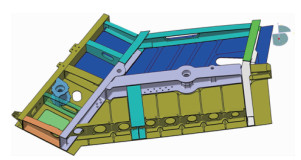图 34 结构组件模型 Fig. 34 Structure componet model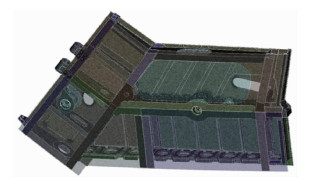图 35 细节应力分析模型 Fig. 35 Detailed stress analysis model
6 内埋武器舱门预紧原理与强度设计 6.1 预紧舱门基本原理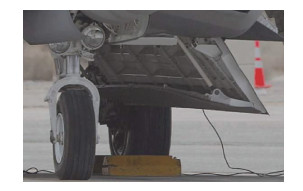图 36 典型预紧内埋武器舱门 Fig. 36 Typical pre-tightening embedded weapon bay door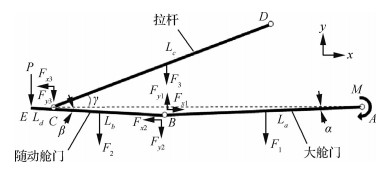图 37 内埋武器舱门结构受力分析 Fig. 37 Structure force analysis of embededded weapon bay door

α+β=0时，

 $m = - Pl + \frac{1}{2}{F_2}(1 - l) + \frac{1}{2}{F_1}$ （12）

α+β≠0时，

 $m = PA + B$ （13）

 $A = \frac{{{\rm{sin}}(\alpha + \beta ){\kern 1pt} {\kern 1pt} {\rm{sin}}\gamma - l{\kern 1pt} {\kern 1pt} {\rm{cos}}(\alpha + \gamma )}}{{{\rm{cos}}(\gamma - \beta )}}$
 $\begin{array}{l} B = \{ {F_1}{\rm{cos}}{\kern 1pt} {\kern 1pt} {\kern 1pt} \alpha {\kern 1pt} {\kern 1pt} {\rm{cos}}(\gamma - \beta ) + \\ {\kern 1pt} {\kern 1pt} {\kern 1pt} {\kern 1pt} {\kern 1pt} {\kern 1pt} {\kern 1pt} {\kern 1pt} {\kern 1pt} {\kern 1pt} {\kern 1pt} {\kern 1pt} {F_2}[2{\rm{sin}}(\alpha + \beta ){\rm{sin}}\gamma + (1 - l){\kern 1pt} {\kern 1pt} {\kern 1pt} {\rm{cos}}{\kern 1pt} {\kern 1pt} \beta {\kern 1pt} {\kern 1pt} {\rm{cos}}(\alpha + \gamma )] + \\ {\kern 1pt} {\kern 1pt} {\kern 1pt} {\kern 1pt} {\kern 1pt} {\kern 1pt} {\kern 1pt} {\kern 1pt} {\kern 1pt} {\kern 1pt} {\kern 1pt} {F_3}{\rm{cos}}{\kern 1pt} \gamma {\kern 1pt} {\kern 1pt} {\rm{sin}}(\alpha + \beta )\} /2{\rm{cos}}(\gamma - \beta ) \end{array}$

1) 只要AB不同时为零，则需要随动舱门与机身支持结构间的接触力P的作用来保持机构的平衡；且所需的把持力矩M与接触力P之间呈线性关系。

2) 当l≤1时，从系数B的表达式可以看出，B>0。因此，如果在该条件下，且P=0，那么则有m=B>0。这说明，在该条件下，即使接触力为零，要保持舱门机构系统的平衡，同样需要施加一定的把持力矩。

3) 当接触力P与系数AB之间存在某种特定关系，即P=-B/A时，可以使得把持力矩m=0。即对于舱门结构系统，如果随动舱门的预紧设计恰当，随动舱门与机体结构间的接触力刚好合适，则舱门结构系统在无把持力矩的条件下也能保持舱门系统本身的平衡。当B>0，即l≤1时，如果A < 0，则必有P>0，即必须存在舱门与机身结构不为零的接触力才能保持舱门结构系统的平衡。

6.2 预紧舱门强度设计关键技术 6.2.1 快速建模技术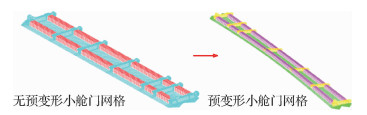图 38 复杂曲面网格快速生成技术应用 Fig. 38 Application of fast generation technology for complex surface mesh
6.2.2 旋转作动器模拟技术

 $\frac{{{M_{\rm{f}}}}}{{{M_{\rm{I}}}}} = \frac{1}{{{\rm{ Ratio }}}} = 1$ （14）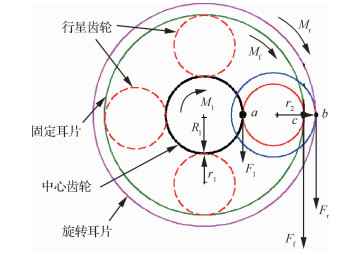图 39 旋转作动器传动原理 Fig. 39 Driving principle of rotary actuator

6.2.3 驱动力矩设计技术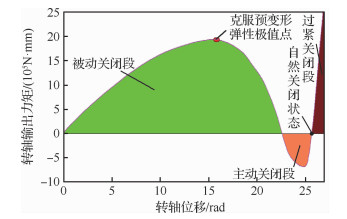图 40 预紧舱门结构的理想驱动力矩曲线 Fig. 40 Ideal driving torque curve of pre-tightened door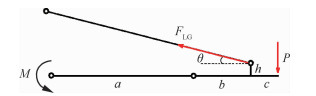图 41 预紧舱门关闭状态简化受力模型 Fig. 41 Brief mechanical model of pre-tightening door in closing state

 $M = Pa\left( {1 - \frac{{b + c}}{{b + h{\rm{cot}}\theta }}} \right)$ （15）

6.2.4 预变形曲率类型及半径设计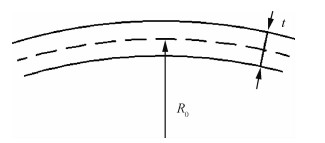图 42 单向曲率预变形构型 Fig. 42 Single curvature of pre-tightening formation

 $\varepsilon = \pm \frac{{\left( {{R_0} + \frac{t}{2}} \right) - {R_0}}}{{{R_0}}} = \pm \frac{t}{{2{R_0}}}$ （16）

 ${R_0} = \frac{t}{{2\varepsilon }} = \frac{{40{\kern 1pt} {\kern 1pt} {\kern 1pt} {\rm{mm}}}}{{2 \times 0.2\% }} = 10{\kern 1pt} {\kern 1pt} {\kern 1pt} 000{\kern 1pt} {\kern 1pt} {\kern 1pt} {\rm{mm}}$
7 双曲面加筋壁板快速建模及声疲劳分析 7.1 双曲面复杂加筋壁板高精度动力有限元建模

7.1.1 模型网格尺寸的确定

 $d = \frac{{{\lambda _{\rm{B}}}}}{6} = \frac{1}{3}{\pi ^4}\sqrt {D + (m{\omega ^2})}$ （17）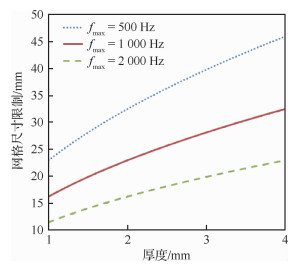图 43 网格尺寸与厚度、最高分析频率的关系 Fig. 43 Relation between mesh size, thickness and maximum analysis frequency

7.1.2 连接件的快速、准确模拟

7.2 有限元/边界元方法求解双曲面结构声振耦合动响应

 $\begin{array}{l} ({\mathit{\boldsymbol{K}}_{\rm{s}}} + {\rm{j}}\omega {\mathit{\boldsymbol{C}}_{\rm{s}}} - {\omega ^2}{\mathit{\boldsymbol{M}}_{\rm{s}}})\{ {u_i}\} + \\ {\kern 1pt} {\kern 1pt} {\kern 1pt} {\kern 1pt} {\kern 1pt} {\kern 1pt} {\kern 1pt} {\kern 1pt} {\kern 1pt} {\kern 1pt} {\kern 1pt} {\kern 1pt} {\kern 1pt} {\kern 1pt} {\kern 1pt} {\kern 1pt} {\kern 1pt} {\kern 1pt} {\kern 1pt} {\mathit{\boldsymbol{K}}_{\rm{c}}}\{ {p_i}\} = \{ {\mathit{\boldsymbol{F}}_{{\rm{s}}i}}\} \end{array}$ （18）

 ${\nabla ^2}p(x,y,z) - {k^2}p(x,y,z) = - {\rm{j}}{\rho _0}\omega q(x,y,z)$ （19）

 $\left[ {\begin{array}{*{20}{c}} {{\mathit{\boldsymbol{K}}_{\rm{s}}} + {\rm{j}}\omega {\mathit{\boldsymbol{C}}_{\rm{s}}} - {\omega ^2}{\mathit{\boldsymbol{M}}_{\rm{s}}}}&{{\mathit{\boldsymbol{L}}_{\rm{s}}}}\\ {{\rho _0}{\omega ^2}\mathit{\boldsymbol{B}}}&\mathit{\boldsymbol{A}} \end{array}} \right] \cdot \left[ {\begin{array}{*{20}{c}} {{u_i}}\\ {{p_i}} \end{array}} \right] = \left[ {\begin{array}{*{20}{c}} {{\mathit{\boldsymbol{F}}_{{\rm{s}}i}}}\\ {{\mathit{\boldsymbol{F}}_{{\rm{a}}i}}} \end{array}} \right]$ （20）

7.3 基于细节模型应力分析的复杂边界应力映射技术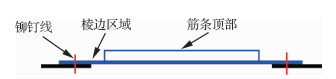图 44 加筋板常见声疲劳裂纹位置 Fig. 44 Location of common acoustic fatigue cracks in stiffened plates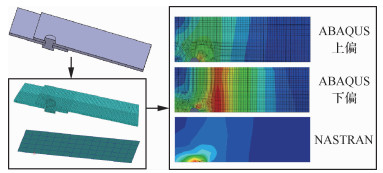图 45 接触和连接对应力的影响 Fig. 45 Effect of contact and connection on stress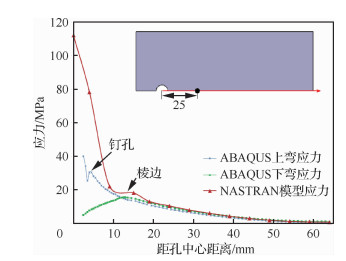图 46 孔边应力 Fig. 46 Stress at hole edge

7.4 双曲面复杂加筋壁板振动疲劳寿命预计

 $n(S) = E(p)Tp(S) = \sqrt {\frac{{{m_4}}}{{{m_2}}}} Tp(S)$ （21）

 ${T_0} = \frac{1}{{E(p)\int_{{S_{{\rm{min}}}}}^{{S_{{\rm{max}}}}} {\frac{{p(S)}}{C}} {S^m}{\rm{d}}S}}$ （22）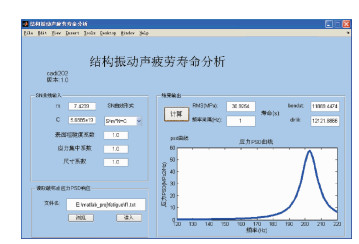图 47 结构振动声疲劳寿命预计程序 Fig. 47 Program for predicting vibration-acoustic fatigue life of structures
8 结构故障预测与健康管理

8.1 SPHM系统

SPHM系统包括在线系统和离线系统。

8.2 SPHM关键技术 8.2.1 SPHM传感器

8.2.2 数据融合与处理

SPHM系统从信号提取、故障检测、诊断和预测、状态评估、决策支持等各个阶段都需要广泛使用数据融合技术，且需在传感器级、特征级、决策级等多个层级上进行，实现多层次、多角度、多参数的检测和诊断以及决策命令的综合智能化。

8.2.3 结构使用载荷/环境谱获取

8.2.4 关键结构疲劳损伤评估

8.2.5 腐蚀损伤/老化预测

8.3 SPHM系统设计

SPHM系统目前采取“在线测量、离线分析”为主的方式，即机载PHM系统实现结构使用载荷/环境有关的数据信息的采集和记录，数据下载到地面系统进行数据的综合处理分析、结构损伤评估和剩余寿命预测等，指导制定结构维修计划。

SPHM机载系统包含机体载荷测量系统，采用成熟的应变电桥测量法获取飞行载荷，应变电桥通过专用胶粘贴在测量位置机体结构上，通过接线端子和连接线组成惠斯通全桥测量电桥，由远程接口单位(RIU)供电和采集数据并由飞管系统存储和管理，概览如图 48所示。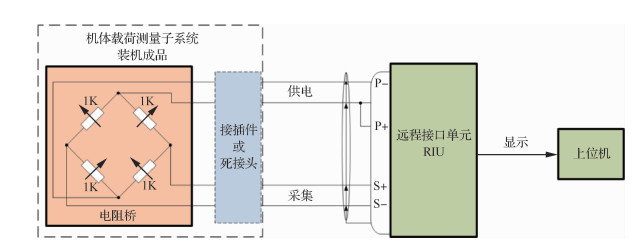图 48 机体载荷监测系统概览图 Fig. 48 Diagram of structure load monitoring system

SPHM地面系统为功能分析软件系统，将机载数据下载到地面系统，结合飞机结构的设计分析资料、试验数据、制造和使用维护信息，对飞机关键结构的疲劳损伤情况进行评估，诊断结构健康状态及预测结构剩余寿命，指导制定结构检查维护计划。技术流程如图 49所示。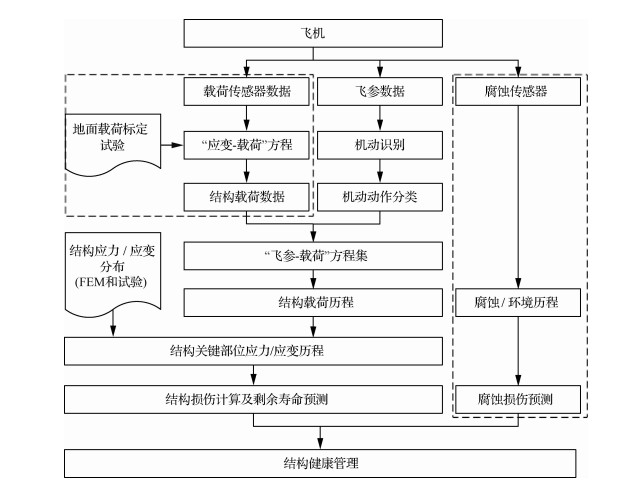图 49 SPHM地面系统技术流程 Fig. 49 Technical flowchart of SPHM off-board system
9 结束语

1) 海量载荷严重工况筛选技术和精度可控的快速分析评估技术。

2) 现代CAE数字技术条件下的总体有限元分析、细节应力分析、工程力学分析、承载能力分析的综合强度设计技术。

3) 基于长期工程设计、分析、试验、使用维护数据的疲劳载荷谱编制与寿命广义类比分析技术。

4) 强度大数据深度分析与综合管理平台建设。

5) 新材料(含复合材料)和新加工工艺的强度分析技术。

6) 结构数字孪生和虚拟试验的研究与应用。

7) 人工智能技术的研究与应用。

http://dx.doi.org/10.7527/S1000-6893.2019.23480

0

#### 文章信息

ZHANG Lixin, ZHONG Shunlu, LIU Xiaodong, FU Huanbing, DUI Hongna, LIU Dongliang, JING Luyun, MOU Binjie, SHI Shanglu

Development and application of strength design technology of high performance fighter

Acta Aeronautica et Astronautica Sinica, 2020, 41(6): 523480.
http://dx.doi.org/10.7527/S1000-6893.2019.23480